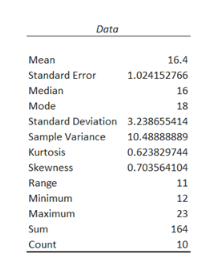# The ages​ (in years) of a random sample of shoppers at a gaming store are shown. Determine the​ range, mean,​ variance, and standard deviation of the sample data set. 1212​,1818​,2323​,1414​,1515​,1818​,1919​,1616​,1313​,1616  The range is1111.​(Simplify your​ answer.)The mean is16.416.4.​(Simplify your answer. Round to the nearest tenth as​ needed.)The variance isnothing.​(Simplify your answer. Round to the nearest hundredth as​ needed.)The standard deviation isnothing.​(Simplify your answer. Round to the nearest tenth as​ needed.)

Question
The ages​ (in years) of a random sample of shoppers at a gaming store are shown. Determine the​ range, mean,​ variance, and standard deviation of the sample data set.

1212​,
1818​,
2323​,
1414​,
1515​,
1818​,
1919​,
1616​,
1313​,
1616

The range is
1111.
The mean is
16.416.4.
The variance is
nothing.
The standard deviation is
nothing.
check_circleExpert Solution
Step 1

Hi, here the data is repeated twice. Hence, consider the below data for further calculations.

Data is 12, 18, 23, 14, 15, 18, 19, 16, 13, 16.

Use the below steps to obtain the​ range, mean,​ variance, and standard deviation by using Excel.

• In Excel sheet, enter Data in one column.
• In Data, select Data Analysis and choose Descriptive Statis...help_outlineImage TranscriptioncloseData 16.4 Mean Standard Error 1.024152766 Median 16 Mode 18 Standard Deviation 3.238655414 Sample Variance 10.48888889 Kurtosis 0.623829744 Skewness 0.703564104 Range 11 Minimum 12 Мaximum 23 Sum 164 Count 10 fullscreen

### Want to see the full answer?

See Solution

#### Want to see this answer and more?

Solutions are written by subject experts who are available 24/7. Questions are typically answered within 1 hour*

See Solution
*Response times may vary by subject and question
Tagged in

### Measures